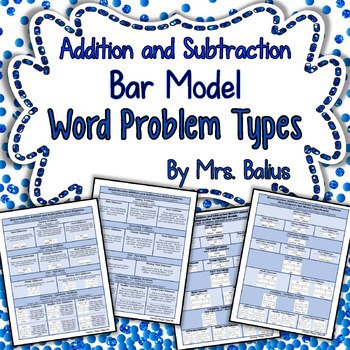# Bar Model Word Problem Types Addition and Subtraction Strip Model Tape ModelSubject
Resource Type
File Type

PDF

(4 MB|4 pages)
Product Rating
Standards
• Product Description
• StandardsNEW
Bar Models, Bar Modeling, Strip Diagrams, Tape Models, Word Problem Types. Addition and Subtraction Word Problems

There are eleven types of addition and subtraction word problems. It is very important that students understand how to set up these problems in order to solve them. This resource is a quick look at the types of problems and the diagrams that may be used to solve them.

Students must be able to write an equation before they complete any mathematical computations for any word problem. Unless students set up the problem correctly and have an appropriate equation, they will not be able to solve for the unknown even if they know the appropriate computational strategies.

This is an expanding resources. I will also be adding other bar modeling tips, tricks, anchor charts and desk resources for addition and subtraction word problems.

It currently includes:

* A pictorial summary of all addition and subtraction bar model types relating to all word problem types. (Two different fonts)
* All word problems types with corresponding word problems for reference.
* A combination of the word problem examples and corresponding bar models for all word problem types.

I use ‘guided math’ in my classroom. I begin my teaching time block teaching/spiraling math concepts as a whole group, but most of my math block is devoted to guided practice in small groups followed by independent or small group practice, centers and intervention. I begin my math time every day with a page from my popular Number of the Day series. You may find more math in this “printable” format in my store.

I hope that you and your kids enjoy this fun back-to-school resource. Check back soon. I continue to add Number of the Day units daily!

Mrs. Balius
++++++++++++++++++++++++++++++++++++++++++
A Whole Year of Number of the Day for First Grade:
Number of the Day {Going Back to School} First Grade Math
Number of the Day {Fall Fun} First Grade Math
Number of the Day {Silly Scary Stuff!} First Grade Math
Number of the Day {Giving Thanks!} First Grade Math November
Number of the Day {Celebrate!} First Grade Math December
Number of the Day {Winter Wonderland} First Grade Math January
Number of the Day {Laissez le Bons Temps Rouler!} First Gr February
Number of the Day {Going to the Rodeo!} First Grade Math March
Number of the Day {Signs of Spring} First Grade Math April
Number of the Day {FIESTA!} First Grade Math May
Number of the Day {Going to the Beach} First Grade Math
Number of the Day {Going Camping!} First Grade Math July

Try this great Counting and Number Line unit:
Counting and Number Lines {Zoo Animal} CCS 1.OA.5 1.NBT.1h

Check out these units in the Number of the Day Series:
Kindergarten:
Purchase the Whole Year here:
Number of the Day {The Whole Year} Kindergarten Bundle

Purchase the whole year here:
Number of the Day {The Whole Year} Second Grade Place Value Bundle

++++++++++++++++++++++++++++++++++++++++++++++++++++++++
Customer Tips:How to get TPT credit to use on future purchases:
• Please go to your My Purchases page (you may need to login). Beside each purchase you'll see a Provide Feedback button. Simply click it and you will be taken to a page where you can give a quick rating and leave a short comment for the product. Each time you give feedback, TPT gives you feedback credits that you use to lower the cost of your future purchases. I value your feedback greatly as it helps me determine which products are most valuable for your classroom so I can create more for you.

Be the first to know about my new discounts, freebies and product launches:
• Look for the green star next to my store logo and click it to become a follower. You will now receive email updates about my store.

Multiply or divide to solve word problems involving multiplicative comparison, e.g., by using drawings and equations with a symbol for the unknown number to represent the problem, distinguishing multiplicative comparison from additive comparison.
Interpret a multiplication equation as a comparison, e.g., interpret 35 = 5 × 7 as a statement that 35 is 5 times as many as 7 and 7 times as many as 5. Represent verbal statements of multiplicative comparisons as multiplication equations.
Use multiplication and division within 100 to solve word problems in situations involving equal groups, arrays, and measurement quantities, e.g., by using drawings and equations with a symbol for the unknown number to represent the problem.
Interpret whole-number quotients of whole numbers, e.g., interpret 56 ÷ 8 as the number of objects in each share when 56 objects are partitioned equally into 8 shares, or as a number of shares when 56 objects are partitioned into equal shares of 8 objects each. For example, describe a context in which a number of shares or a number of groups can be expressed as 56 ÷ 8.
Interpret products of whole numbers, e.g., interpret 5 × 7 as the total number of objects in 5 groups of 7 objects each. For example, describe a context in which a total number of objects can be expressed as 5 × 7.
Total Pages
4 pages
N/A
Teaching Duration
N/A
Report this Resource to TpT
Reported resources will be reviewed by our team. Report this resource to let us know if this resource violates TpT’s content guidelines.